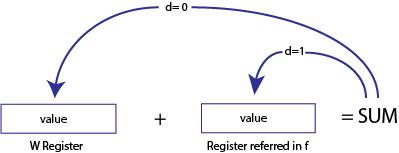Add the contents of the W register with register ‘f’. If ‘d’ is ‘0’, the result is stored in the W register. If ‘d’ is ‘1’, the result is stored back in register ‘f’.

Syntax:

Operation:Operands:

• f is from 0 to 127 (decimal)
• d is either 0 or 1

Status Affected: C, DC, Z

Example:

```ADDWF REG, 0
```

Before instruction:

```W  = 0x20
REG = 0x01
```

After instruction:

```W = 0x21
REG = 0x01```

Example:

`ADDWF REG, 1`

Before instruction:

```W = 0x20
REG = 0x01
```

After instruction:

```W = 0x20
REG = 0x21```